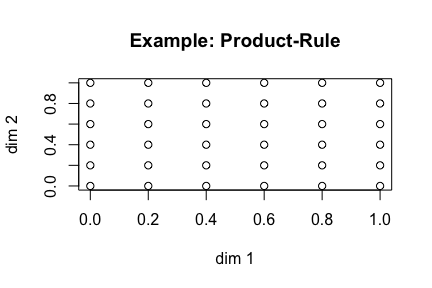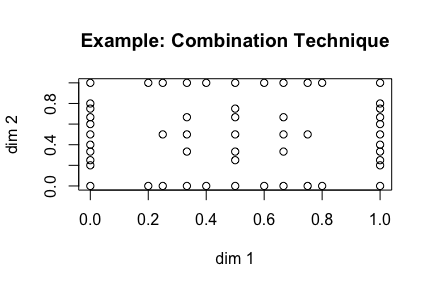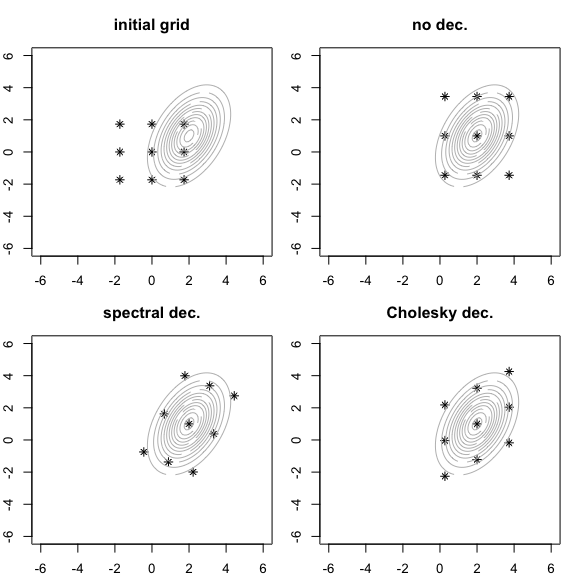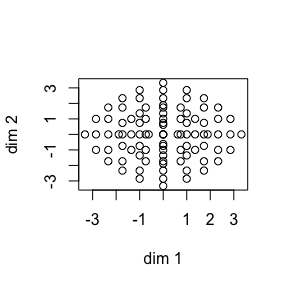## Introduction

This package provides a collection of methods for (potentially) multivariate quadrature in R. It’s especially designed for use in statistical problems, characterized by integrals of the form $$\int_a^b g(x)p(x) \ dx$$, where $$p(x)$$ denotes a density-function. Furthermore the methods are also applicable to standard integration problems with finite, semi-finite and infinite intervals.

In general quadrature (also named: numerical integration, numerical quadrature) work this way: The integral of interests is approximated by a weighted sum of function values.

$I = \int_a^b h(x) \ dx \approx A = \sum_{i=1}^n w_i \cdot h(x_i)$

The so called nodes ($$x_i$$) and weights($$w_i$$) are defined by the chosen quadrature rule, which should be appropriate (better: optimal) for the integration problem in hand1.

This principle can be transferred directly to the multivariate case.

The methods provided in this package cover the following tasks:

• creating an uni-/multivariate grid (grid: collection of nodes and weights) for a chosen quadrature rule
• examining the created grid
• rescaling the grid for appropriate/efficient use
• computing the approximated integral

## Quick Start

This section shows a typical workflow for quadrature with the mvQuad-package. More details and additional features of this package are provided in the subsequent sections. In this illustration the following two-dimensional integral will be approximated: $I = \int_1^2 \int_1^2 x \cdot e^y \ dx dy$

  library(mvQuad)

# create grid
nw <- createNIGrid(dim=2, type="GLe", level=6)

# rescale grid for desired domain
rescale(nw, domain = matrix(c(1, 1, 2, 2), ncol=2))

# define the integrand
myFun2d <- function(x){
x[,1]*exp(x[,2])
}

# compute the approximated value of the integral
A <- quadrature(myFun2d, grid = nw)

Explanation Step-by-Step

1. mvQuad-package is loaded
2. with the createNIGrid-command a two-dimensional (dim=2) grid, based on Gauss-Legendre quadrature rule (type="GLe") with a given accuracy level (level=6) is created and stored in the variable nw

The grid created above is designed for the domain $$[0, 1]^2$$ but we need one for the domain $$[1, 2]^2$$

1. the command rescale changes the domain-feature of the grid; the new domain is passed in a matrix (domain=...)

2. the integrand is defined

3. the quadrature-command computes the weighted sum of function values as mentioned in the introduction

## Supported Rules (build in)

The choice of quadrature rule is heavily related to the integration problem. Especially the domain/support ($$[a, b]$$ (finite), $$[a, \infty)$$ (semi-finite), $$(-\infty, \infty)$$ (infinite)) determines the choice.

The mvQuad-packages provides the following quadrature rules.

• cNC1, cNC2, ..., cNC6: closed Newton-Cotes Formulas of degree 1-6 (1=trapezoidal-rule; 2=Simpson’s-rule; …), finite domain

• oNC0, oNC1, ..., oNC3: open Newton-Cote Formula of degree 0-3 (0=midpoint-rule; …), finite domain

• GLe, GKr: Gauss-Legendre and Gauss-Kronrod rule, finite domain

• nLe: nested Gauss-Legendre rule (finite domain) (Petras 2003)

• Leja: Leja-Points (finite domain)

• GLa: Gauss-Laguerre rule (semi-finite domain)

• GHe: Gauss-Hermite rule (infinite domain)

• nHe: nested Gauss-Hermite rule (infinite domain) (Genz and Keister 1996)

• GHN, nHN: (nested) Gauss-Hermite rule as before but weights are pre-multiplied by the standard normal density ($$\hat{w}_i = w_i * \phi(x_i)$$).2

For each rule grids can be created of different accuracy. The adjusting screw in the createNIGrid is the level-option. In general, the higher level the more precise the approximation. For the Newton-Cotes rules an arbitrary level can be chosen. The other rules uses lookup-tables for the nodes and weights and are therefore restricted to a maximum level (see help(QuadRules))

## User-defined Rules

Through the very general functionality of quadrature, mvQuad allows to use user-defined quadrature rules. This can be of interest for specific integration problems.

There are two ways to hand over user-defined rules to createNIGrid. (1) Via a function, which takes a value for the level and returns a grid. The returned grid have to be a list with three objects (n: nodes, w: weights, features: initial domain; see the example below). (2) Via a text-file, which contains the nodes and weights for potentially several levels.

Variant 1

# via an user-defined function
myRule.fun <- function(l){
n <- seq(1, 2*l-1, by=2)/ (l*2)
w <- rep(1/(l), l)

initial.domain <- matrix(c(0,1), ncol=2)
return(list(n=as.matrix(n), w=as.matrix(w), features=list(initial.domain=initial.domain)))
}

nw.fun <- createNIGrid(d=1, type = "myRule.fun", level = 10)
## Warning in seq(1, 2 * l - 1, by = 2)/(l * 2): Recycling-Array der Länge 1 in Vektor-Array-Rechnungen ist veraltet.
##  Bitte stattdessen c() oder as.vector() nutzen.
  print(data.frame(nodes=getNodes(nw.fun), weights=getWeights(nw.fun)))
##    nodes weights
## 1   0.05     0.1
## 2   0.15     0.1
## 3   0.25     0.1
## 4   0.35     0.1
## 5   0.45     0.1
## 6   0.55     0.1
## 7   0.65     0.1
## 8   0.75     0.1
## 9   0.85     0.1
## 10  0.95     0.1

Variant 2

# via a text-file
nw.txt <- createNIGrid(d=1, type = myRule.txt, level = 10)
print(data.frame(nodes=getNodes(nw.txt), weights=getWeights(nw.txt)))
##    nodes weights
## 1   0.05     0.1
## 2   0.15     0.1
## 3   0.25     0.1
## 4   0.35     0.1
## 5   0.45     0.1
## 6   0.55     0.1
## 7   0.65     0.1
## 8   0.75     0.1
## 9   0.85     0.1
## 10  0.95     0.1

The text-file for variant 2 have to be formatted like the following example. The first line have to declare the domain initial.domain a b, where a and b denotes the lower and upper-bound for the integration domain. This can be either a number or ‘-Inf’/‘Inf’ (for example initial.domain 0 1 or initial.domain 0 Inf)

Every following line contains one single node and weight belonging to one level of the rule (format: ‘level’ ‘node’ ‘weight’). This example shows the use for the “midpoint-rule” (levels: 1 - 3).

## 1     initial.domain 0 1
## 2     1 0.5 1
## 3     2 0.25 0.5
## 4     2 0.75 0.5
## 5     3 0.166666666666667 0.333333333333333
## 6     3 0.5 0.333333333333333
## 7     3 0.833333333333333 0.333333333333333
## 8     4 0.125 0.25
## 9     4 0.375 0.25
##    ...

## Construction of multivariate grids

The quadrature rules described above are inherent 1-dimensional. There exists several construction principles for multivariate grids, based on 1D-quadrature rules. The traditional one is the so called “product-rule”, which leads to an even designed grid (see figure “Product-Rule”). The main drawback of this principle is the fast growing number of grid points for an increasing number of dimensions. The total number of grid points $$N = n^d$$, where $$n$$ denotes the number of points in the underlying 1d-rule. This property make this principle unfeasible for higher dimensions (d>4).

createNIGrid can be set to use this principle with the ndConstruction="product" argument

  nw <- createNIGrid(dim=2, type="cNC1", level=5, ndConstruction = "product")
plot(nw, main="Example: Product-Rule")Another principle is the “combination technique”, which leads to an more sophisticated compilation of grid points. Therefore the construction is a bit more complex Heiss and Winschel (2008), but the number of grid points grow much slower with increasing dimensionality, with almost the same accuracy. Therefore sparse grids are feasible for more then 20 dimensions creatNIGrid can be forced to use this principle with the ndConstruction=“sparse” argument.

  nw <- createNIGrid(dim=2, type="cNC1", level=5, ndConstruction = "sparse")
plot(nw, main="Example: Combination Technique")## Rescaling

createNIGrid generate an un-adjusted grid. Un-adjusted in the sense that it maybe doesn’t fit the domain needed for the integration problem in hand or it is not efficient. Typically build in grids for finite intervals are adjusted for $$[0, 1]^d$$ and grids for infinite intervals for a density with zero mean and variance equals one.

A first example for rescaling was shown in the Quick-Start section. Another one is shown here. A bivariate normal density with mean-vector $$m=(2,1)'$$ and covariance matrix $$C=\begin{pmatrix} 1.0 & 0.6 \\ 0.6 & 2.0 \end{pmatrix}$$ should be integrated for $$[-\infty, \infty]^2$$.

The following four figures shows the adjusted grid added by the contour plot of the integrand.The upper left figure shows the initial grid (optimal for zero mean an variance equals 1). The upper right figure shows a rescaled grid, where for the mean and variances (diagonal elements of $$C$$) is accounted for. In both lower pictures it’s also accounted for the covariance, left via the spectral-decomposition, right via the Cholesky decomposition (Jäckel 2005).

Here is the code for the rescaling:

  nw <- createNIGrid(dim=2, type="GHe", level=3)

# no rescaling
plot(nw, main="initial grid", xlim=c(-6,6), ylim=c(-6,6), pch=8)

rescale(nw, m = m, C = C, dec.type = 0)
plot(nw, main="no dec.", xlim=c(-6,6), ylim=c(-6,6), pch=8)

rescale(nw, m = m, C = C, dec.type = 1)
plot(nw, main="spectral dec.", xlim=c(-6,6), ylim=c(-6,6), pch=8)

rescale(nw, m = m, C = C, dec.type = 2)
plot(nw, main="Cholesky dec.", xlim=c(-6,6), ylim=c(-6,6), pch=8)

## Examinig the Grid

For technical or didactic reasons there is sometimes the need to examine a created grid. The mvQuad-package provides the methods illustrated in the following example-session

  nw <- createNIGrid(dim=2, type="GHe", level=6, ndConstruction = "sparse")

plot(nw)print(nw)
## Grid for Numerical Integration
##  # dimensions: 2  # gridpoints: 91    used memory:2.7 Kb
##
## nD-Construction principle:  'sparse'
##  same quadrature rule for each dimension
##       type:  GHe
##       level:  6
##       initial domain:  -Inf Inf
  dim(nw)
##  2
  size(nw)
## $dim ##  2 ## ##$gridpoints
##  91
##
## \$memory
## 2792 bytes
##
## attr(,"class")
##  "NIGrid.size"

## Miscellaneous

1. What mvQuad (V. 1.0.1) can’t do:
• mvQuad can’t select an appropriate quadrature rule for your problem.
• The grid create by ‘createNIGrid’ is static. There is no refinement nor adaption.
• There is no error estimate, which gives you a feeling of the achieved accuracy.
1. Save created grids. The creation of high-dimensional grids is ‘time-consuming’. Ones you are satisfied by an grid for a concrete task, save the grid (i.e. save(nw, file=....)`) for replications.

2. Ex ante it’s not trivial to find a balance between ‘standard grids’ and ‘highly adapted grids’. Both approaches ‘brute force’ and ‘high specialization’ can cost a lot of CPU- and/or live-time.

3. All comments regarding the package or the documentation are highly appreciated.

1. A rigorous introduction to numerical integration can be found in Davis/Rabinowitz (1984)↩︎

2. Those rules are computationally more efficent for integrands of the form: $$\int_{-\infty}^{\infty} g(x)\phi(x)dx$$ because the approximation reduces to $$\sum \hat{w}_i \cdot g(x)$$.↩︎